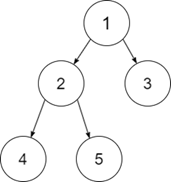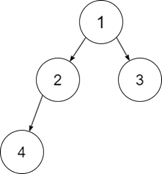# C++ Program to Check Whether a given Binary Tree is a Full Binary Tree or not

Given a Binary Tree, the task is to check whether it is a Full Binary Tree or not. A Binary Tree is said to be a Full Binary Tree if every node has zero or two children.

For Example

Input-1Output:

1

Explanation: Every node except the leaf node has two children, so it is a full binary tree.

Input-2:Output:

0

Explanation: Node 2 has only one child, so it is not a full binary tree.

## Approach to Solve this Problem

To check whether a given binary tree is full or not, we can check recursively for the left subtree and right subtree.

• Input a given Binary Tree having nodes and its children.
• A Boolean function isFullBinaryTree(Node*root) takes the root node as the input and returns True if it is full binary tree, otherwise false.
• In the base condition, if the root node is NULL or empty, then return True.
• If the left subtree and the right subtree is NULL or empty, then return True.
• Now let us check recursively for each of the left subtree and right subtree and return the output.

## Example

Live Demo

#include<iostream>
using namespace std;
struct treenode {
int data;
treenode * left;
treenode * right;
};
struct treenode * createNode(int d) {
struct treenode * root = new treenode;
root -> data = d;
root -> left = NULL;
root -> right = NULL;
return root;
}
bool isFullBinaryTree(struct treenode * root) {
if (root == NULL) {
return true;
}
if (root -> left == NULL and root -> right == NULL) {
return true;
} else if (root -> left and root -> right) {
return (isFullBinaryTree(root -> left) and isFullBinaryTree(root -> right));
}
return false;
}
int main() {
struct treenode * root = NULL;
root = createNode(1);
root -> left = createNode(2);
root -> right = createNode(3);
root -> left -> right = createNode(4);
root -> left -> left = createNode(5);
root -> right -> left = createNode(6);
if (isFullBinaryTree(root)) {
cout << "1" << endl;
} else {
cout << "0" << endl;
}
return 0;
}

Running the above code will generate the output as,

## Output

0

Explanation: Since all the leaf nodes in the given binary tree do not have children nodes, it is not a Full Binary Tree. So, we get the output as 0.